## What we undergrad students (at least I did for…

What we undergrad students (at least I did for a long time) take for granted is that Bell’s inequality proofs there is for sure no deterministic theory of quantum mechanics. Is it really that “easy”? Maybe it’s pretty naive to accept the Copenhagen Interpretation without further thinking. Tim Maudlin who studied philosophy and physics at Yale University explains why we should consider less conventional “interpretations” of quantum physics.

You can find his corresponding paper here.

## Regular

Brian Greene is going to do this new video series from tomorrow onwards – I think it’s worth it to check it out 🙂

Btw do you know why Brian Greene is an extraordinary cool physicist? Because he’s vegan 😉

## How does one get this idea [for the proof]? Th…

How does one get this idea [for the proof]? The answer is: I don’t know! It is like asking: How did Michelangelo do this?

Nice

## Happy Pi Day to the math fellows out there!Happy Pi Day to the math fellows out there!

## This One Equation, 10² + 11² + 12² = 13² + 14²…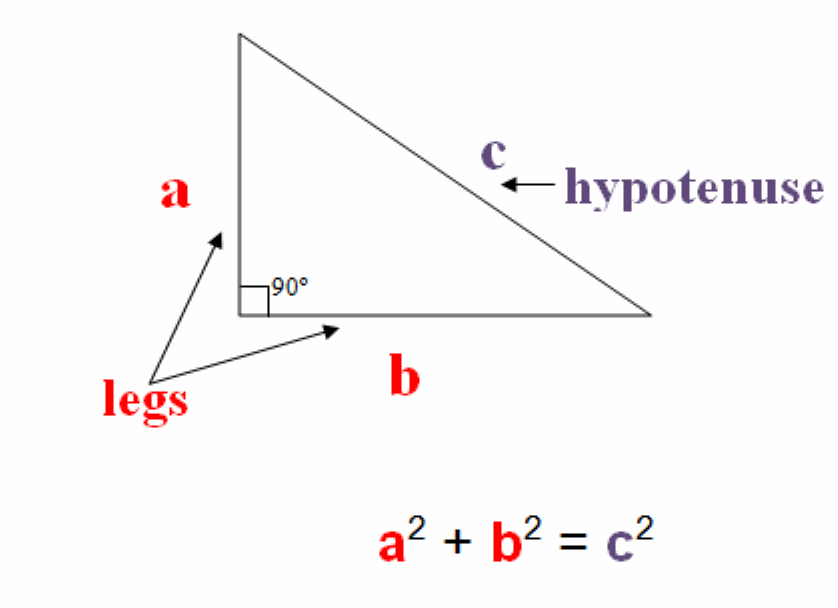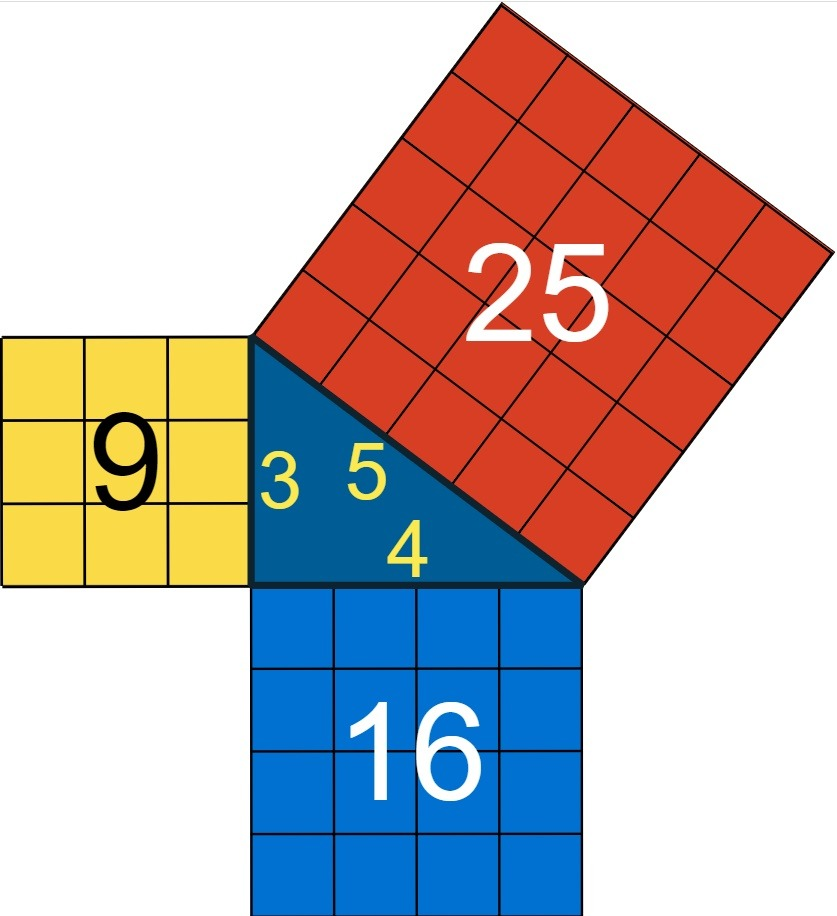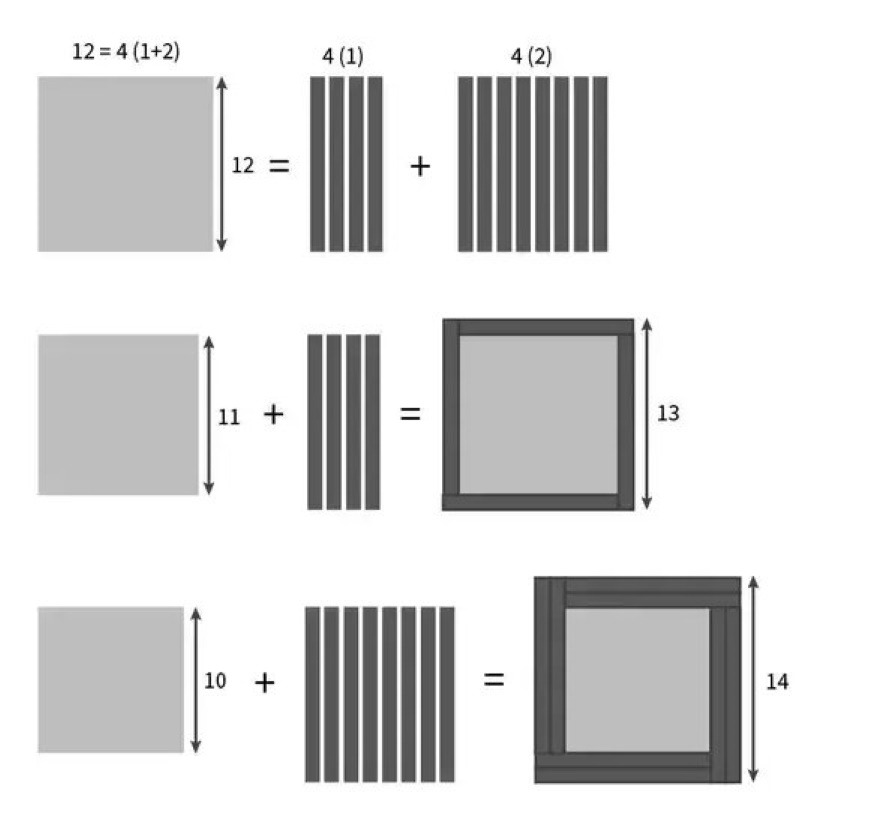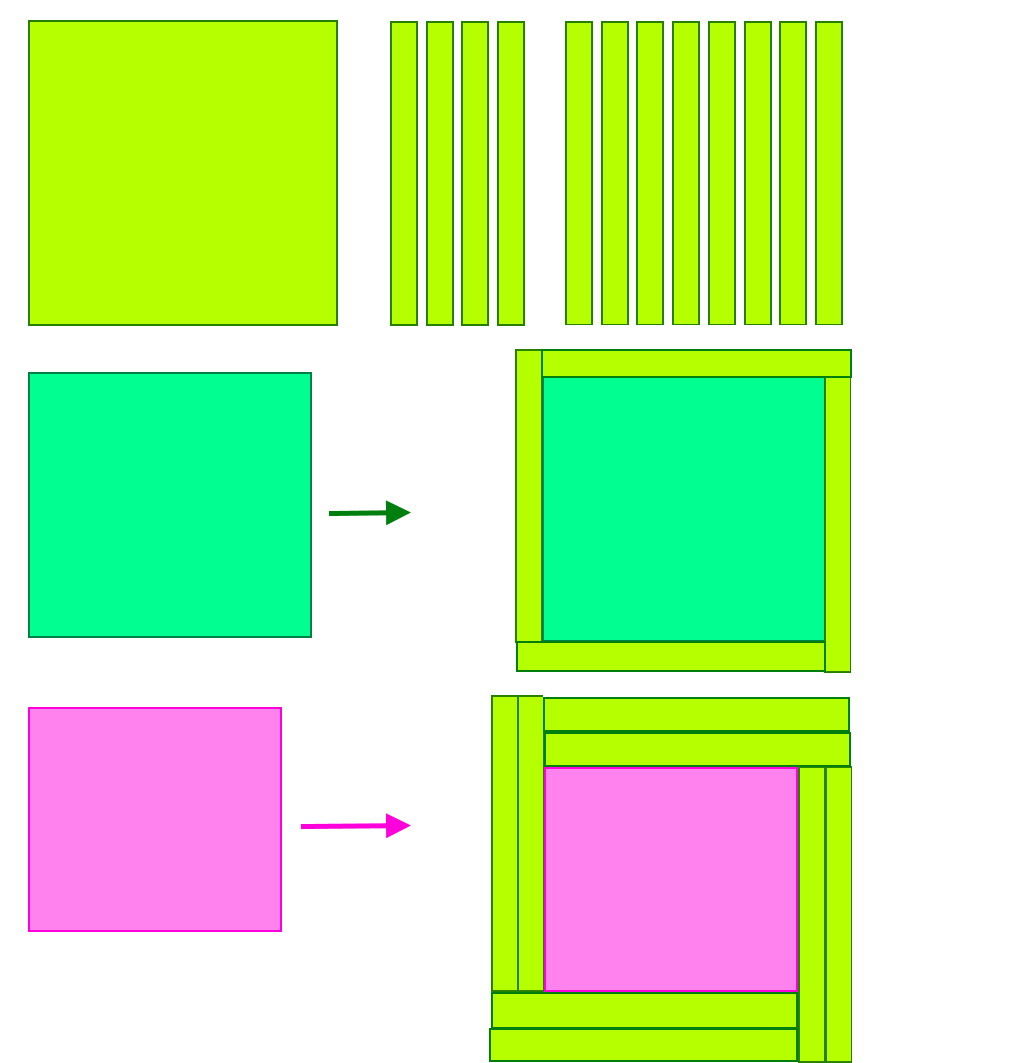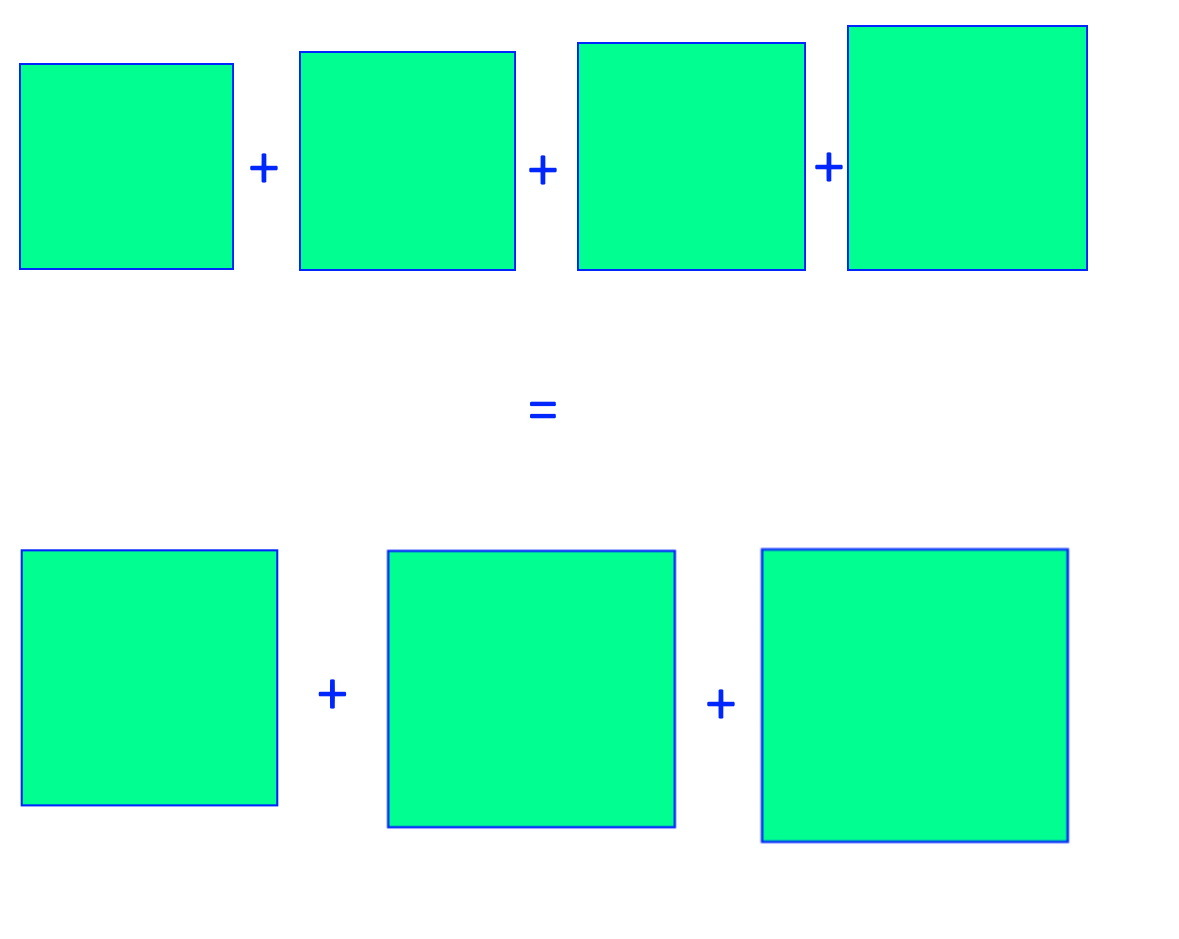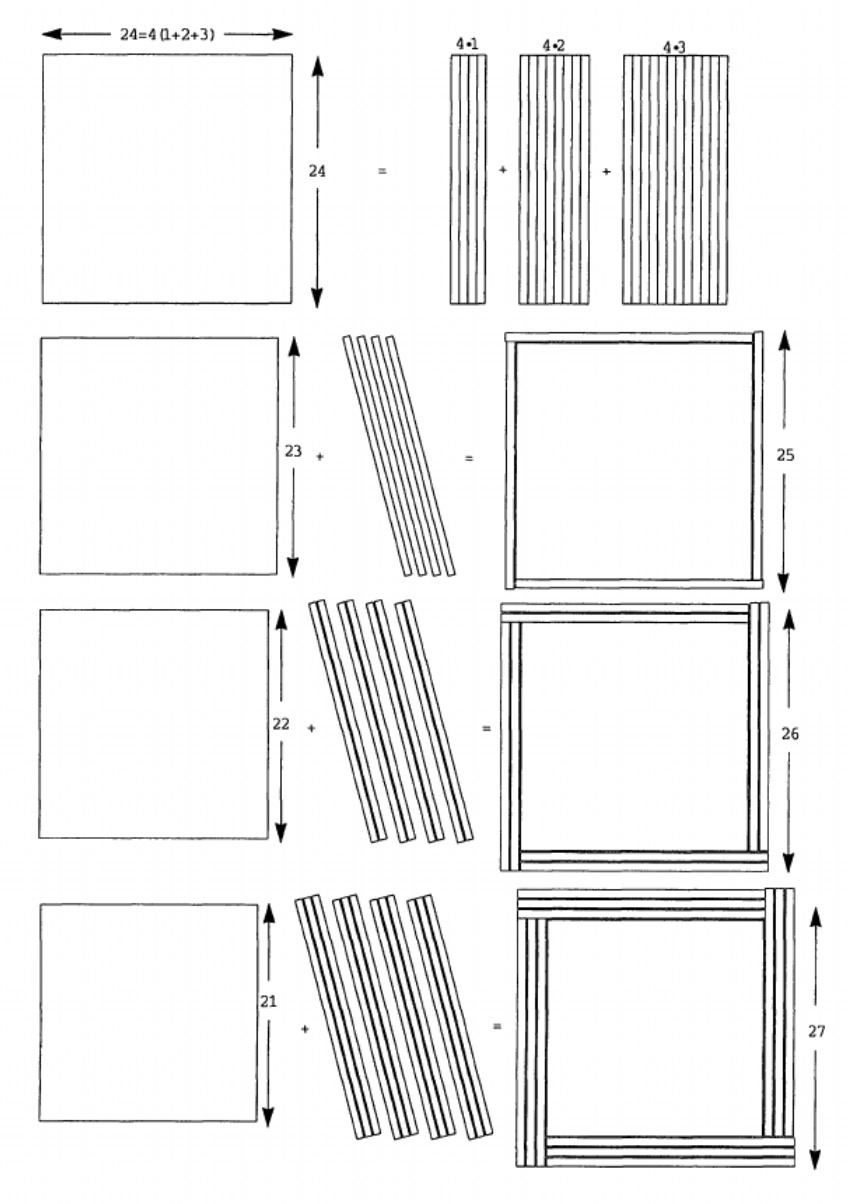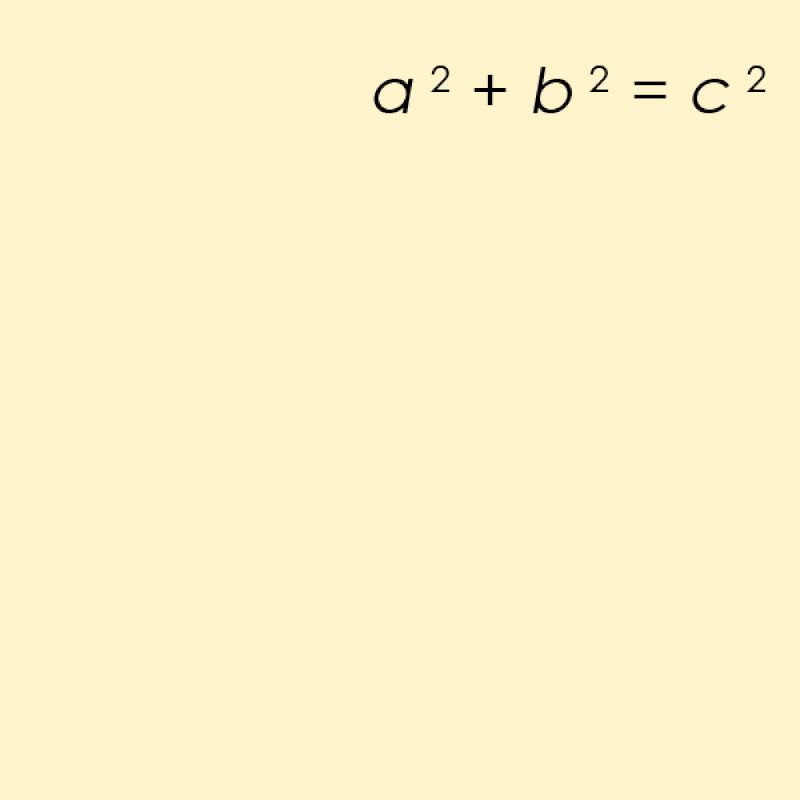## This One Equation, 10² + 11² + 12² = 13² + 14², Takes Pythagoras To A Whole New Level

“There are 365 days in a (non-leap) year, and 10² + 11² + 12² = 13² + 14² = 365. However, this mathematical fact doesn’t have anything to do with our calendar at all, nor with our planet’s rotation and revolution around the Sun. Instead, the number of days in a year is pure coincidence here, but the mathematical relation is a direct consequence of Pythagorean geometry, something far easier to visualize than just algebra.

Pythagoras just started with a² + b² = c², which has 3, 4, and 5 as the only set of consecutive numbers that solve it. We can extend this as long as we like, however, and for each equation with an odd number of terms we can write down, there’s only one unique solution of consecutive whole numbers. These Pythagorean Runs have a clever mathematical structure governing them, and by understanding how squares work, we can see why they couldn’t possibly behave in any other way.”

You’ve likely seen the Pythagorean Theorem before, which is about the sides and hypotenuses of a right triangle. The only solution of consecutive whole numbers to it is 3² + 4² = 5², which is maybe the simplest right triangle you can imagine. There may not be other consecutive number solutions to this problem, but if you consider having consecutive strings of more numbers, like 5, 7, or any odd number of them, there’s always one unique solution you can find, and they all follow a fascinating pattern.

It’s one of those mathematical instances where sure, you can solve it using algebra, but it’s beautiful and easy to see using geometry. Come enjoy the math of Pythagorean Runs today!

## Tomorrow is my quantum physics exam – instead …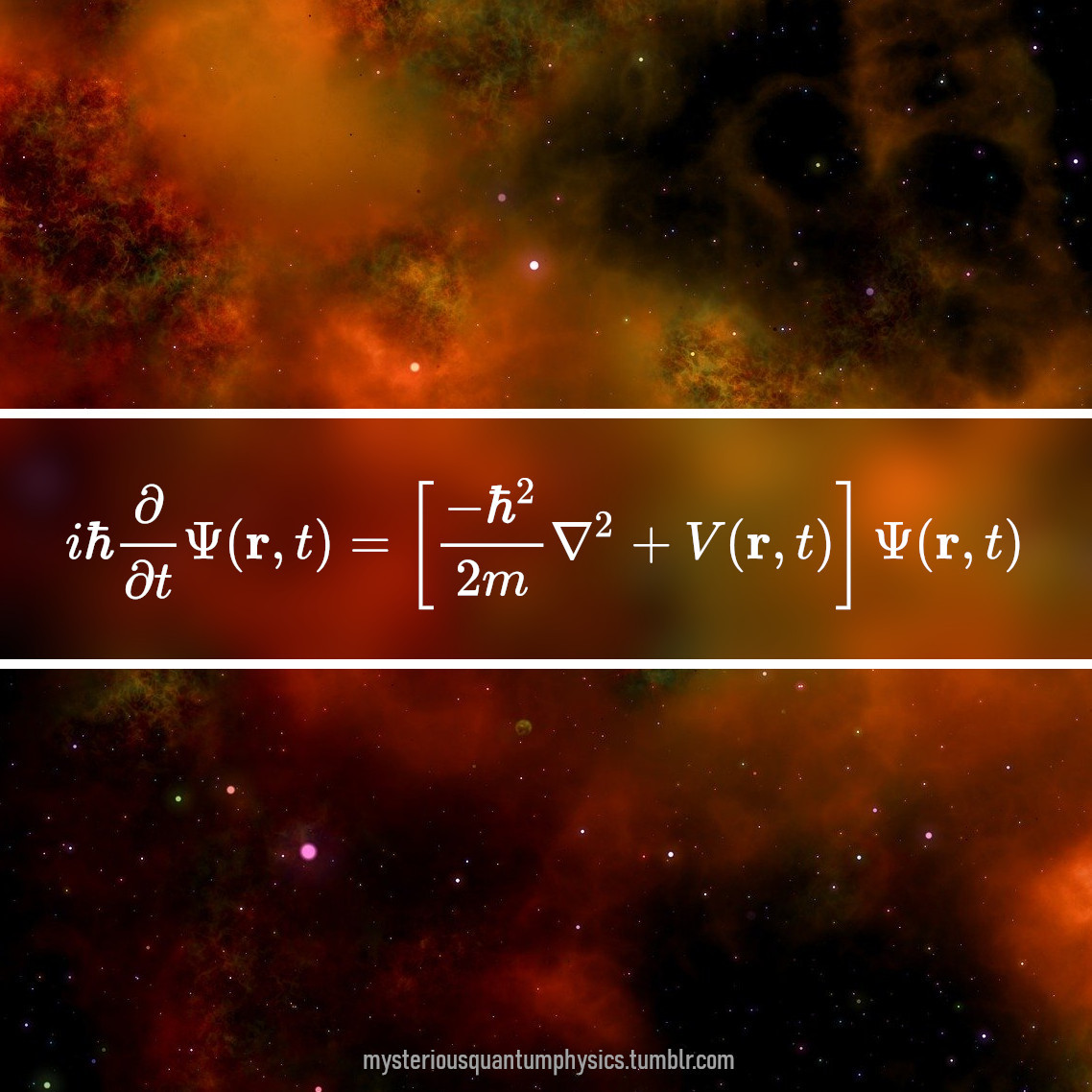Tomorrow is my quantum physics exam – instead of panicking I try to embrace the beauty of the time dependent Schrödinger equation in position space.

## 2020 is a leap year and here’s a inforgraphic that illustrates…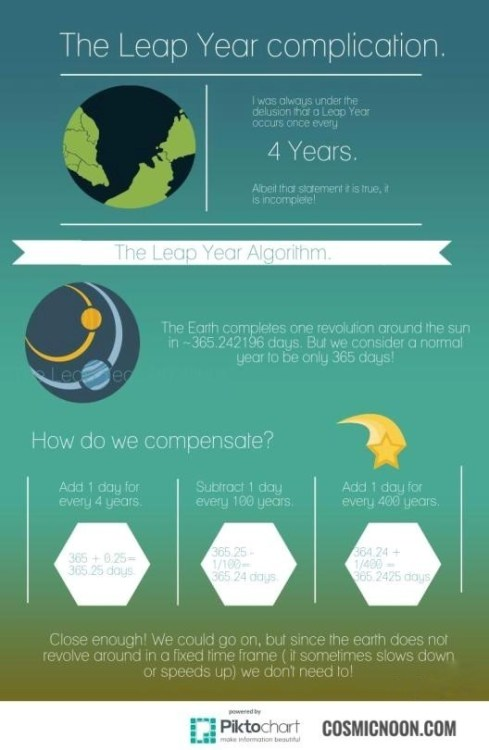2020 is a leap year and here’s a inforgraphic that illustrates how the ‘leap year algorithm’ works.

## Regular

“Mathematics is the taming of the infinite.”

— Linear Algebra professor

## Regular

Everything we call real is made of things that cannot be regarded as real.

Niels Bohr